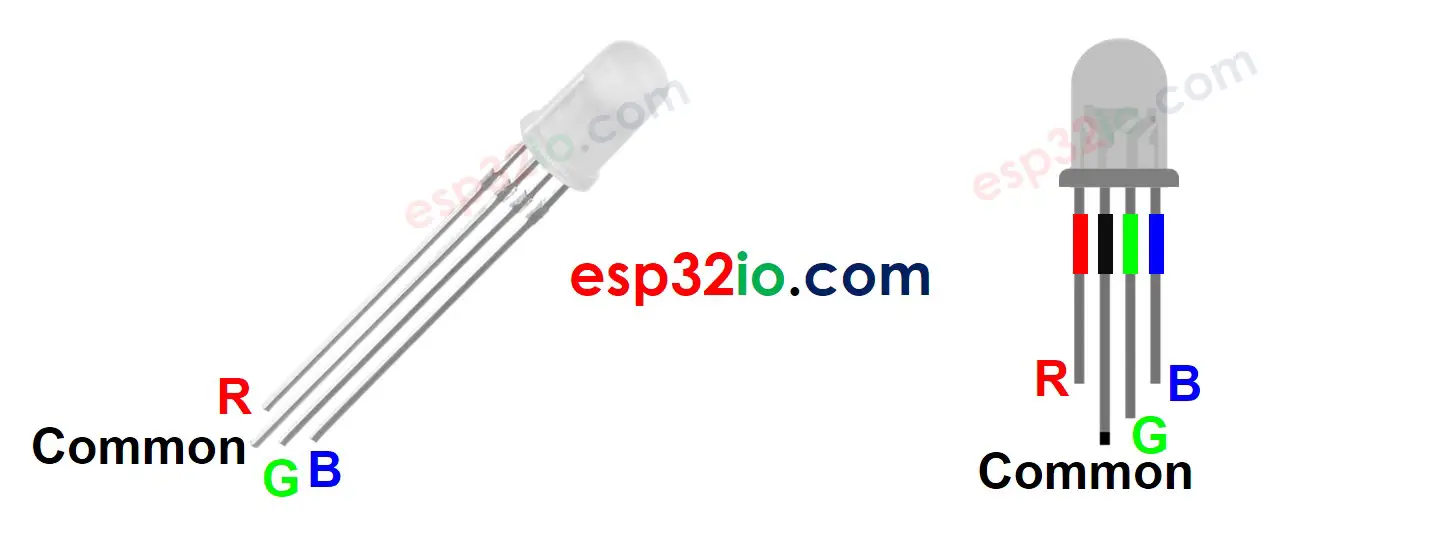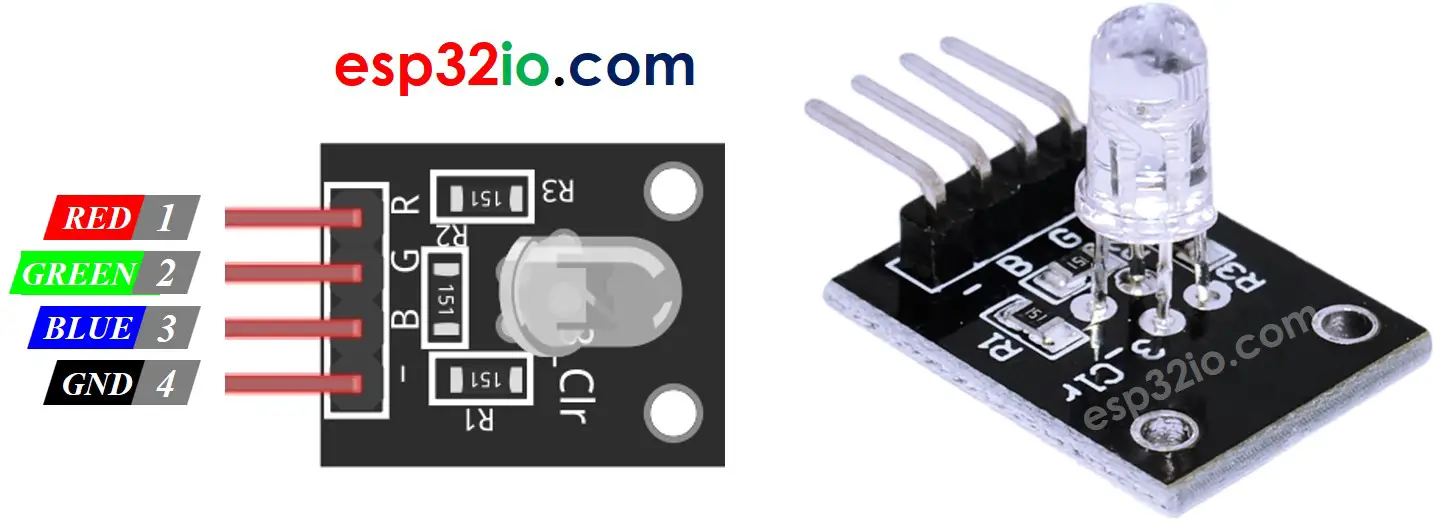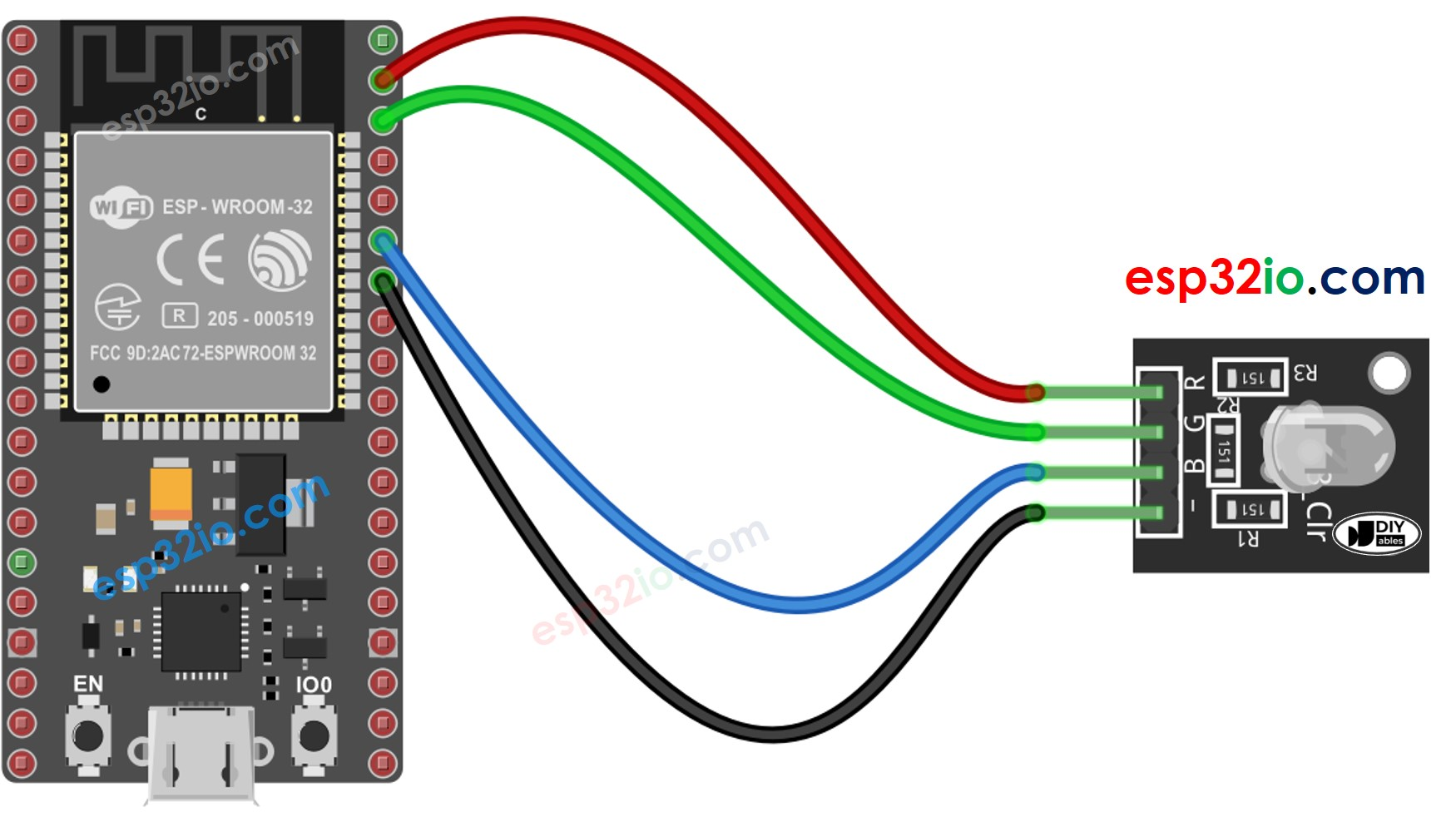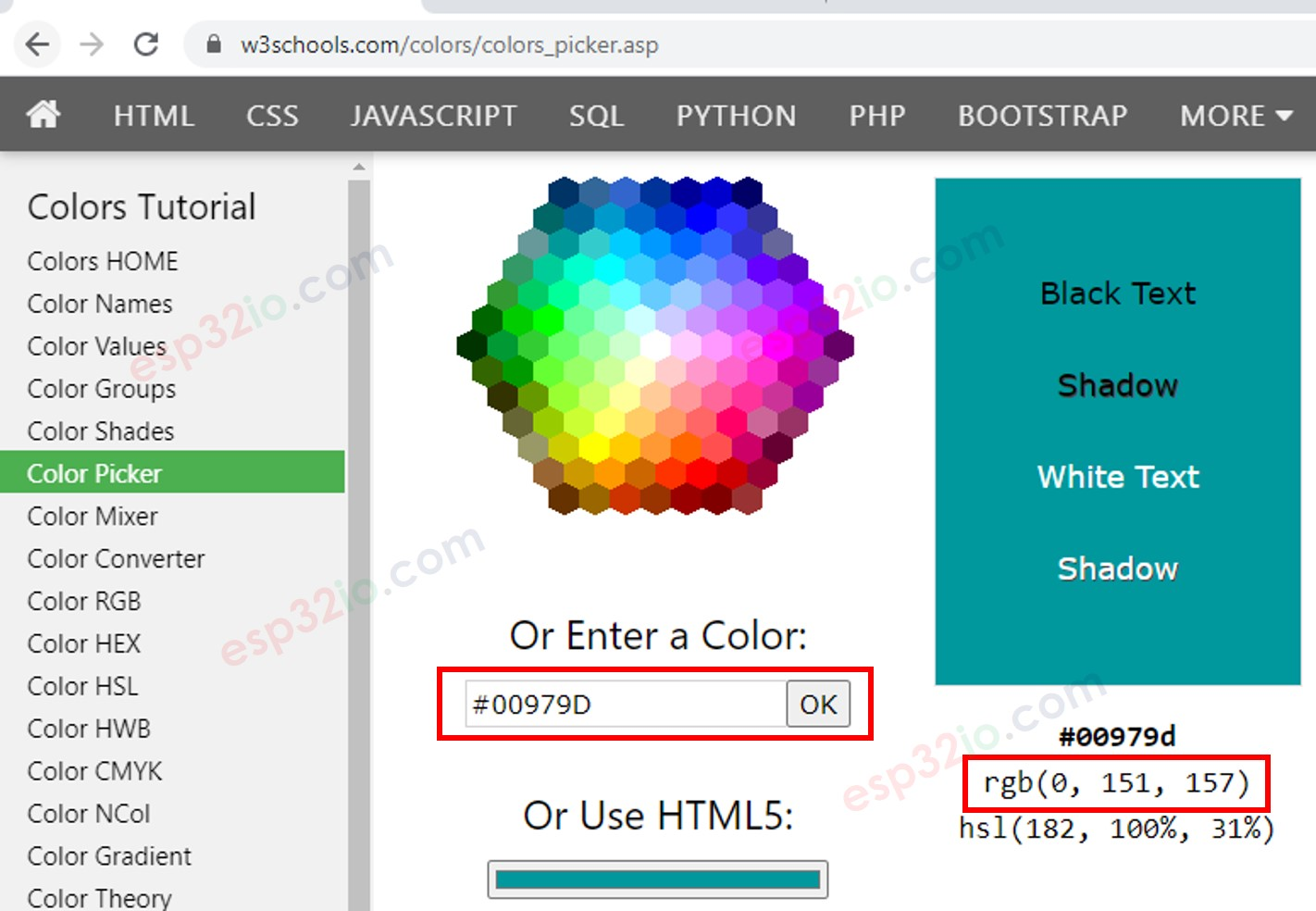# ESP32 - RGB LED

This tutorial instructs you how to control RGB LED to emit any color using ESP32.

## Hardware Used In This Tutorial

Or you can buy the following sensor kit:

Disclosure: some of these links are affiliate links. We may earn a commission on your purchase at no extra cost to you. We appreciate it.

## Introduction to RGB LED

The RGB LED can emit any colors by mixing the 3 basic colors red, green and blue. A single RGB LED is composed of 3 LEDs: red, green and blue. These three LEDs are packed into a single case so that it looks like a single LED.

### RGB LED Pinout

RGB LED includes four pins:

• R (red) pin: is to control the red color element
• G (green) pin: is to control the green color element
• B (blue) pin: is to control the blue color element
• Common (Cathode-) pin: connect this pin to GND (0V)To hook up RGB LED to ESP32, we gotta add current-limiting resistors. This can complicate the wiring. Luckily, we can use an RGB LED module that comes with pre-built current-limiting resistors.

RGB LED Module also includes four pins:

• Common (Cathode-) pin: needs to be connected to GND (0V)
• R (red): pin is used to control red
• G (green): pin is used to control green
• B (blue): pin is used to control blue※ NOTE THAT:

According to the common pin, there are two types of LED: common anode and common cathode. This tutorial uses a common cathode LED.

### How RGB LED works

In term of physics, a color is a combination of three color elements: Red (R), Green (G) and Blue (B). Each color element's value range is from 0 to 255. The combination of values of three color elements create 256 x 256 x 256 colors in total.

If we generate PWM signals to R, G, B pins, the RGB LED diplays a color corresponding to the PWM duty cycle values. By changing the duty cycle of PWM signals (from 0 to 255), the RGB LED can display any color. The color values of Red (R), Grean (G) and Blue (B) correspond to PWM duty cycle on R, G and B pins , respectively.

## Wiring Diagram between RGB LED and ESP32

• Wiring diagram between ESP32 and RGB LEDThis image is created using Fritzing. Click to enlarge image

• Wiring diagram between ESP32 and RGB LED moduleThis image is created using Fritzing. Click to enlarge image

## How To Control RGB LED

Let's assume that we want to display #00979D color on RGB LED, we can do the following step:

• Find the color code. Tips:
• Convert color code to R, G, B values using the tool from w3school. Take note these values. in this case: R = 0, G = 151, B = 157• Define ESP32 pins that connects to R, G, and B pins. For example:
#define PIN_RED 23 // GPIO23 #define PIN_GREEN 22 // GPIO22 #define PIN_BLUE 21 // GPIO21
• Configure these ESP32 pins to the output mode
pinMode(PIN_RED, OUTPUT); pinMode(PIN_GREEN, OUTPUT); pinMode(PIN_BLUE, OUTPUT);
• Control LED to emit that color (#00979D → R = 0, G = 151, B = 157)
analogWrite(PIN_RED, 0); analogWrite(PIN_GREEN, 151); analogWrite(PIN_BLUE, 157);

## ESP32 - RGB LED Example Code

The below code changes color of LED among following colors in sequence:

• #00C9CC (R = 0, G = 201, B = 204)
• #F7788A (R = 247, G = 120, B = 138)
• #34A853 (R = 52, G = 168, B = 83)
/* * This ESP32 code is created by esp32io.com * * This ESP32 code is released in the public domain * * For more detail (instruction and wiring diagram), visit https://esp32io.com/tutorials/esp32-rgb-led */ #define PIN_RED 23 // GPIO23 #define PIN_GREEN 22 // GPIO22 #define PIN_BLUE 21 // GPIO21 void setup() { pinMode(PIN_RED, OUTPUT); pinMode(PIN_GREEN, OUTPUT); pinMode(PIN_BLUE, OUTPUT); } void loop() { // color code #00C9CC (R = 0, G = 201, B = 204) analogWrite(PIN_RED, 0); analogWrite(PIN_GREEN, 201); analogWrite(PIN_BLUE, 204); delay(1000); // keep the color 1 second // color code #F7788A (R = 247, G = 120, B = 138) analogWrite(PIN_RED, 247); analogWrite(PIN_GREEN, 120); analogWrite(PIN_BLUE, 138); delay(1000); // keep the color 1 second // color code #34A853 (R = 52, G = 168, B = 83) analogWrite(PIN_RED, 52); analogWrite(PIN_GREEN, 168); analogWrite(PIN_BLUE, 83); delay(1000); // keep the color 1 second }

#### When using many colors, we could shorten the code by creating a function:

/* * This ESP32 code is created by esp32io.com * * This ESP32 code is released in the public domain * * For more detail (instruction and wiring diagram), visit https://esp32io.com/tutorials/esp32-rgb-led */ #define PIN_RED 23 // GPIO23 #define PIN_GREEN 22 // GPIO22 #define PIN_BLUE 21 // GPIO21 void setup() { pinMode(PIN_RED, OUTPUT); pinMode(PIN_GREEN, OUTPUT); pinMode(PIN_BLUE, OUTPUT); } void loop() { // color code #00C9CC (R = 0, G = 201, B = 204) setColor(0, 201, 204); delay(1000); // keep the color 1 second // color code #F7788A (R = 247, G = 120, B = 138) setColor(247, 120, 138); delay(1000); // keep the color 1 second // color code #34A853 (R = 52, G = 168, B = 83) setColor(52, 168, 83); delay(1000); // keep the color 1 second } void setColor(int R, int G, int B) { analogWrite(PIN_RED, R); analogWrite(PIN_GREEN, G); analogWrite(PIN_BLUE, B); }

※ OUR MESSAGES